Previous: 6.1.2 Hydrodynamical Formulation Up: 6.1 Quantum Mechanics in Next: 6.2 Quantum Mechanics in

## 6.1.3 The Riccati and Prüfer Equation

The Riccati equation was used in [Bit00] as a numerically more suitable variant of the hydrodynamical formulation.

Set(6.28)

With this the stationary Schrödinger equation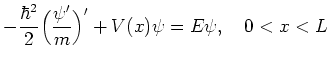becomes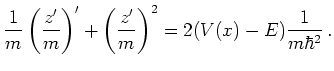(6.29)

By the substitution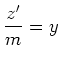(6.30)

it reduces to a nonlinear first order equation. For constant mass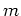and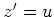we get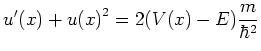(6.31)

This type of equation is known as Riccati equation [MR96]. The Riccati equation plays an important role in control theory and has a rich theory of its own. Separation of Equation 6.30 into real and imaginary part recovers the hydrodynamical equation for the phase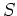as equation for the imaginary part of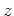.

Another alternative formulation of the Schrödinger equation, which is closely related to the Riccati equation, is the Prüfer equation. The Prüfer transformation is a useful tool in the qualitative theory of second order Sturm-Liouville differential equations [BD01]. In the case of the Schrödinger equation with constant massit is introduced in the following way: Define complex quantities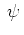(6.32)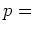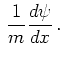(6.33)

Introducing the transformation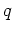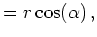(6.34)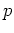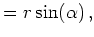(6.35)

with complex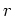, and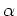we get from the Schrödinger equation and from the defining equation for(6.34)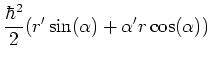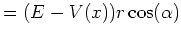(6.36)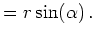(6.37)

Respective multiplication of these equations with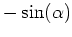and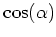and adding the resulting equations yields (after elimination of):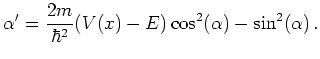(6.38)

So the equation for the angular variable separates. Forwe get: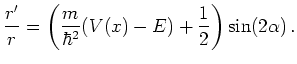(6.39)

The link to the original Riccati idea is immediate. Ifis a solution to the Prüfer equation, then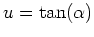is a solution to the Riccati Equation 6.32. The advantage of the Prüfer equation (6.39) over the traditional Riccati equation is that it can be solved for arbitrarywithout leading to singularities, as is the case with nodes (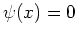) in the Riccati equation. The Schrödinger, Riccati and Prüfer equation will be investigated numerically in Section 7.1.3.